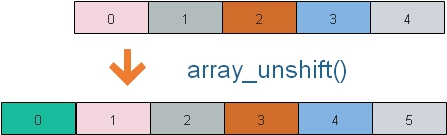# array_unshift(): Adding one element at starting of the array

``\$output=array_unshift(\$input);``To add one or more element at the beginning of the array. After applying `array_unshift()` total elements of the array increases by one or more . All numerical array keys are re-indexed but literal keys remain same.

Returns the total number of elements present (after adding using array_unshift() to the array.)

Here \$input is an array, \$output get the value of total number of elements of the array.
``````\$input=array('One','Two','Three','Four','Five');
echo \$output; // Output is  6
echo "<br><br>";
while (list (\$key, \$val) = each (\$input)) {
echo "\$key -> \$val <br>";
}``````
Output of above code is here( \$output gets the number of elements present 6 )
``````6

1 -> One
2 -> Two
3 -> Three
4 -> Four
5 -> Five``````
To add more than one element we will change the code like this.
``\$output=array_unshift(\$input,'added_one','add_two');``

## With Literal Keys

``````\$input=array('Fruits1' =>'Banana','Fruits2'=>'Mango','Fruits3'=>'Apple','Fruits4'=>'Grapes');
\$output=array_unshift(\$input, 'Strawberry');
echo \$output; // Output is 5
echo "<br><br>";
while (list (\$key, \$val) = each (\$input)) {
echo "\$key -> \$val <br>";
}``````
Output is here, ( \$output gets the first element Banana)
``````5

0 -> Strawberry
Fruits1 -> Banana
Fruits2 -> Mango
Fruits3 -> Apple
Fruits4 -> Grapes``````
Now key of the first element is 0. To add one element with key to an associative array we have to use the code below.
``````\$input=array('Fruits1' =>'Banana','Fruits2'=>'Mango','Fruits3'=>'Apple','Fruits4'=>'Grapes');
\$input=array('new_fruit'=> 'Strawberry') + \$input;
while (list (\$key, \$val) = each (\$input)) {
echo "\$key -> \$val <br>";
}``````
Output is here
``````new_fruit -> Strawberry
Fruits1 -> Banana
Fruits2 -> Mango
Fruits3 -> Apple
Fruits4 -> Grapes``````

## Using current

By using current() we can get the element to which the present internal pointer is pointing. By defalut it points to first element of the array.
``````\$input=array('Fruits1' =>'Banana','Fruits2'=>'Mango','Fruits3'=>'Apple','Fruits4'=>'Grapes');
echo current(\$input);
echo "<br>";
\$output=array_unshift(\$input,'Strawberry');
echo current(\$input); ``````
Output is here.( The first element is changed from Banana to Mango)
``````Banana
Strawberry``````

Subscribe to our YouTube Channel here

## Subscribe

* indicates required
Subscribe to plus2netplus2net.com

Post your comments , suggestion , error , requirements etc here .